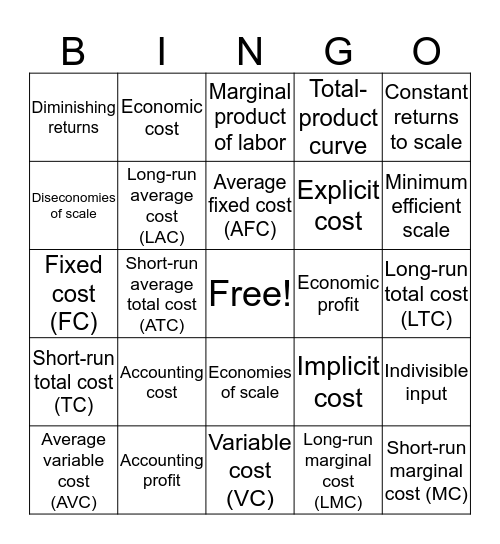# BUS3200 Microeconomics - Chapter 8This bingo card has a free space and 24 words: Accounting cost, Accounting profit, Average fixed cost (AFC), Average variable cost (AVC), Constant returns to scale, Diminishing returns, Diseconomies of scale, Economic cost, Economic profit, Economies of scale, Explicit cost, Fixed cost (FC), Implicit cost, Indivisible input, Long-run average cost (LAC), Long-run marginal cost (LMC), Long-run total cost (LTC), Marginal product of labor, Minimum efficient scale, Short-run average total cost (ATC), Short-run marginal cost (MC), Short-run total cost (TC), Total-product curve and Variable cost (VC).

## Play Online